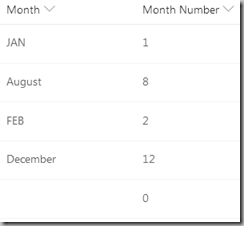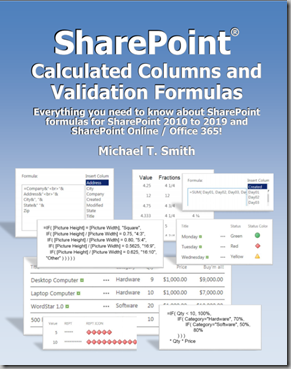## 10/30/2018

### SharePoint Calculated Columns – Convert a Month Name to a Number

Here are three solutions for when you have a column with a three letter abbreviation of a month name, and you want the month number,

Each of the following assume that you have a "Single Line of Text" or "Choice" column named "Month".

### A long IF statement:

```IF([Month]="JAN",1,
IF([Month]="FEB",2,
IF([Month]="MAR",3,
IF([Month]="APR",4,
IF([Month]="MAY",5,
IF([Month]="JUN",6,
IF([Month]="JUL",7,
IF([Month]="AUG",8,
IF([Month]="SEP",9,
IF([Month]="OCT",10,
IF([Month]="NOV",11,
IF([Month]="DEC",12,
0
))))))))))))```

### Using the FIND() Function:

```=(
FIND(
UPPER(D2),
"   ,JAN,FEB,MAR,APR,MAY,JUN,JUL,AUG,SEP,OCT,NOV,DEC")
) -1
) / 4```

FIND returns the position of the matching text. "JAN" returns five. If we subtract one and then divide the FIND number by four, we get the month number.

### Finding the Month Number Using Three or More Month Letters

```=(
FIND(
UPPER(Month),
"         ,JANUARY  ,FEBRUARY ,MARCH    ,APRIL    ,MAY      ,JUNE     ,JULY     ,AUGUST   ,SEPTEMBER,OCTOBER  ,NOVEMBER ,DECEMBER "
)  -1
) / 10```

This uses the same idea, but the month names are spelled out and are padded with spaces to make each one nine characters long. (Ten with the commas.)

Here’s the result:Now available on Amazon!